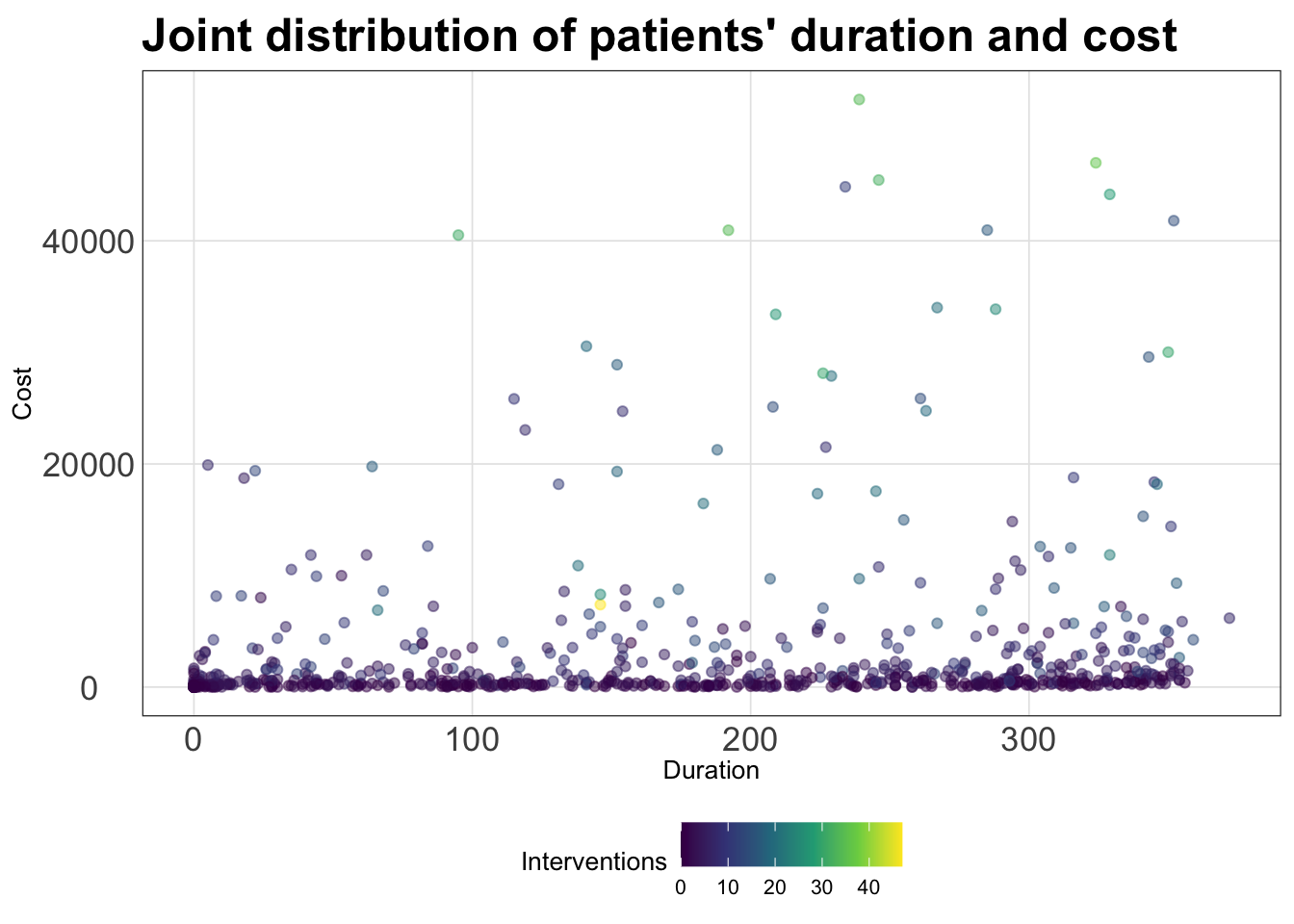Released on Monday, June 12, 2023

Let’s start again by reading in the data from last lab using the `read_csv()` function after loading the `tidyverse`:

``````library(tidyverse)

# SPORTS

# HEALTH

The NBA dataset contains information about players from the 2022 season.

The heart disease dataset was collected by a health insurance company gathering information on subscribers who had claims resulting from ischemic (coronary) heart disease. The dataset contains total costs and types of services for one year. The variables in this dataset include:

Variable Description
Cost Total cost of subscriber claims
Age Age of claimant
Gender Gender of claimant
Interventions Number of interventions / procedures given to patient
Drugs Factor describing number of drugs prescribed to patient
ERVisit Number of ER visits
Complications Factor describing number of complications experienced by the patient
Comorbidities Number of comorbidities the patient had
Duration Duration of treatment
id Index variable

## Peeking at the data

Write code that displays the column names of your dataset (`nba_stats` or `heart_disease`). Also, look at the first six rows of your dataset to get an idea of what these variables look like. Which variables are quantitative, and which are categorical?

``# INSERT CODE HERE``

#### (SPORTS)

The NBA dataset has no indicator variables, so it doesn’t need any further processing at this time. (But feel free to check out the health section to see how we would deal with that)

#### (HEALTH)

As it turns out, even though `Drugs` and `Complications` appear to be quantitative - they are actually categorical variables. Specifically, `Drugs` represents the categorized number of drugs prescribed: 0 if none, 1 if one, 2 if more than one; `Complications` indicates whether or not the subscriber had complications: 1 if yes, 0 if no. To address this issue for our plots, we can manually recode the variables as factors. For instance, we can modify the `Complications` variable using a simple if-else statement:

``````heart_disease <- heart_disease %>%
mutate(Complications = ifelse(Complications == 0, "No", "Yes"))``````

This is a quick fix to the binary indicator variable since, by default, `R` orders factor variables in alphabetical order. In this case, “No” is before “Yes” because “N” is before “Y”. We may not want variables in alphabetical order however - we will see how to change this in lecture.

Next, to update the `Drugs` variable we will use the `fct_recode()` function which allows us to manually change the labels of a factor variable:

``````heart_disease <- heart_disease %>%
mutate(Drugs = fct_recode(as.factor(Drugs),
"None" = "0", "One" = "1", "More than one" = "2"))``````

Why did we have to specify `as.factor(Drugs)` first then place the numbers in quotation marks?

## Always make a bar chart…

Now we’ll use the `ggplot()` function to create a bar plot of one of the categorical variables. To make things easier, we provide the code for you to do this below; just uncomment the code and run it to create the bar plot. In what follows, you must answer some questions about the code and plot.

#### (SPORTS)

Make a bar plot of `position` in the NBA dataset.

``````# Create the bar plot of position:
# nba_stats %>%
#   ggplot(aes(x = position)) +
#   geom_bar(fill = "darkblue") +
#   labs(title = "Number of NBA players by position",
#        x = "Position", y = "Number of players",

#### (HEALTH)

Make a bar plot of `Drugs` in the heart disease dataset.

``````# Create the bar plot of Drugs:
# heart_disease %>%
#   ggplot(aes(x = Drugs)) +
#   geom_bar(fill = "darkblue") +
#   labs(title = "Number of patients by number of drugs",
#        x = "Number of drugs", y = "Number of patients")``````

#### (ALL TOGETHER NOW)

• In general, `ggplot()` code takes the following format: `ggplot(blank1, aes(x = blank2))`. Looking at the above code, what kind of `R` object should `blank1` be, and what should `blank2` be?

• What do you think the line `geom_bar(fill = "darkblue")` does?

• What do you think the remaining lines of code do (contained in `labs()`)?

## More area plots (but bar plots are better!)

Now we’ll make a few other area plots:

• spine chart

• pie chart

• rose diagram

Your goal for this part is to create each of these plots. These plots can be created by copy-and-pasting the bar plot code from above and modifying it slightly. Follow these directions to create each of these plots:

• spine chart: First, copy-and-paste the bar plot code from above. Then, delete the `fill = "darkblue"` within `geom_bar()`. Finally, within `ggplot()`, replace `aes(x = position)` with `aes(x = "", fill = position)` (SPORTS), or replace `aes(x = Drugs)` with `aes(x = "", fill = Drugs)` (HEALTH). Also, change the labels in `labs()` if necessary.
``# PUT YOUR SPINE CHART CODE HERE``
• pie chart: First, copy-and-paste the spine chart code you just made. Then, after `geom_bar()`, “add” `coord_polar("y")`. Be sure to put plus signs before and after `coord_polar("y")`. Also, change the labels in `labs()` if necessary.
``# PUT YOUR PIE CHART CODE HERE``
• rose diagram: First, copy-and-paste your original bar plot code. Then, after `geom_bar(fill = "darkblue")`, “add” `coord_polar() + scale_y_sqrt()`. Be sure to put plus signs before and after `coord_polar() + scale_y_sqrt()`. Also, change the labels in `labs()` if necessary. After you make the rose diagram: In 1-2 sentences, what do you think `scale_y_sqrt()` does, and what is a benefit to including `scale_y_sqrt()` when making the rose diagram?
``# PUT YOUR ROSE DIAGRAM CODE HERE``

## Notes on colors in plots

Three types of color scales to work with:

1. Qualitative: distinguishing discrete items that don’t have an order (nominal categorical). Colors should be distinct and equal with none standing out unless otherwise desired for emphasis.
• Do NOT use a discrete scale on a continuous variable
1. Sequential: when data values are mapped to one shade, e.g., for an ordered categorical variable or low to high continuous variable
• Do NOT use a sequential scale on an unordered variable
1. Divergent: think of it as two sequential scales with a natural midpoint midpoint could represent 0 (assuming +/- values) or 50% if your data spans the full scale
• Do NOT use a divergent scale on data without natural midpoint

### Options for `ggplot2` colors

The default color scheme is pretty bad to put it bluntly, but `ggplot2` has ColorBrewer built in which makes it easy to customize your color scales.

#### (SPORTS)

For instance, we can make a scatterplot with `three_pointers` on the y-axis and `offensive_rebounds` on the x-axis and using the `geom_point()` layer with each point colored by `position`:

``````nba_stats %>%
ggplot(aes(x = offensive_rebounds, y = three_pointers,
color = position)) +
geom_point(alpha = 0.5) +
labs(x = "Offensive rebounds", y = "Three-pointers",
color = "Position") +
theme_bw()``````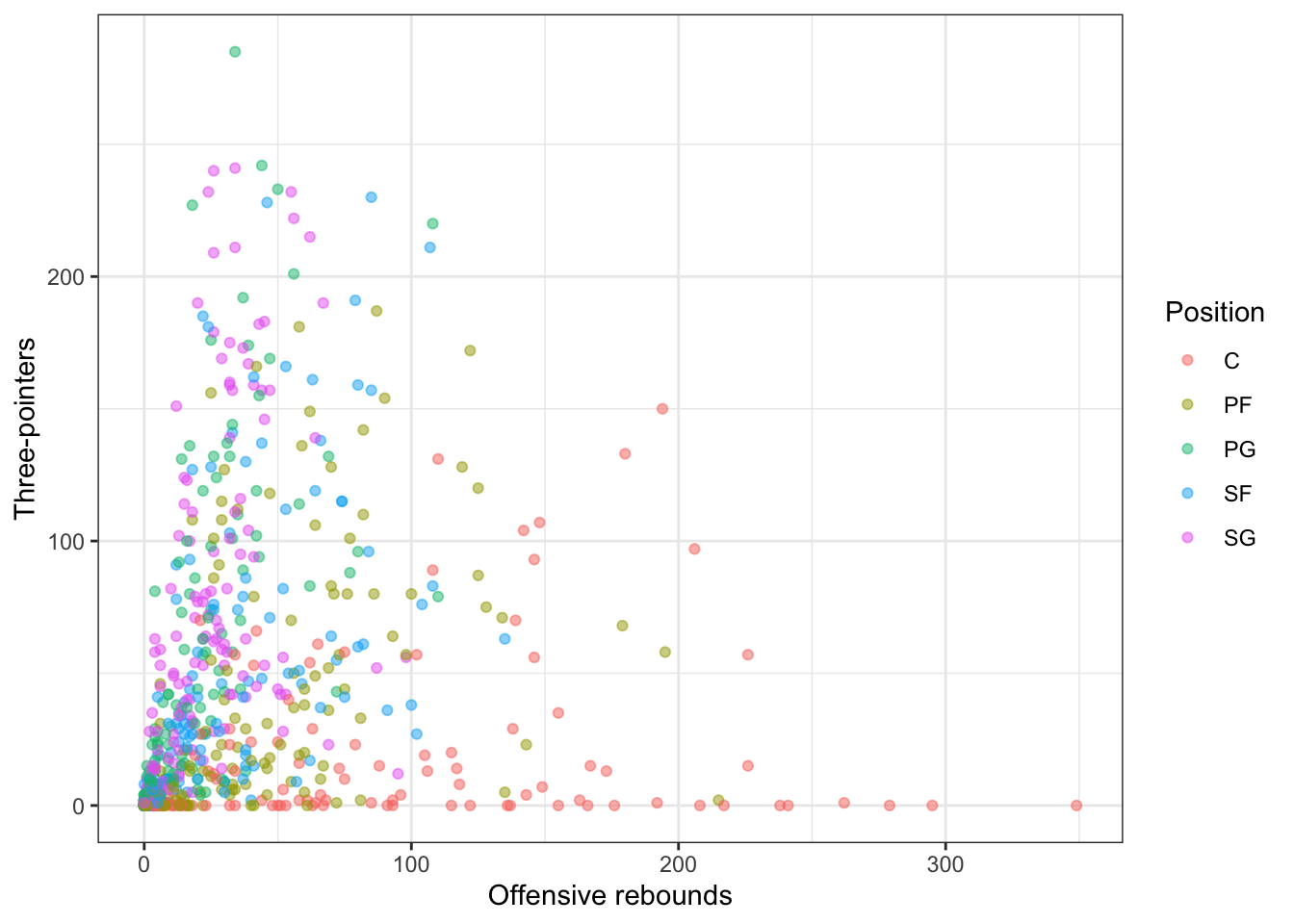What does `alpha` change? We can change the color plot for this plot using `scale_color_brewer()` function:

``````nba_stats %>%
ggplot(aes(x = offensive_rebounds, y = three_pointers,
color = position)) +
geom_point(alpha = 0.5) +
scale_color_brewer(palette = "Set2") +
labs(x = "Offensive rebounds", y = "Three-pointers",
color = "Position") +
theme_bw()``````#### (HEALTH)

Alternatively, we can make a scatterplot with `Cost` on the y-axis and `Duration` on the x-axis and using the `geom_point()` layer with each point colored by `Drugs`:

``````heart_disease %>%
ggplot(aes(x = Duration, y = Cost,
color = Drugs)) +
geom_point(alpha = 0.5) +
labs(x = "Duration", y = "Cost",
color = "Number of drugs") +
theme_bw()``````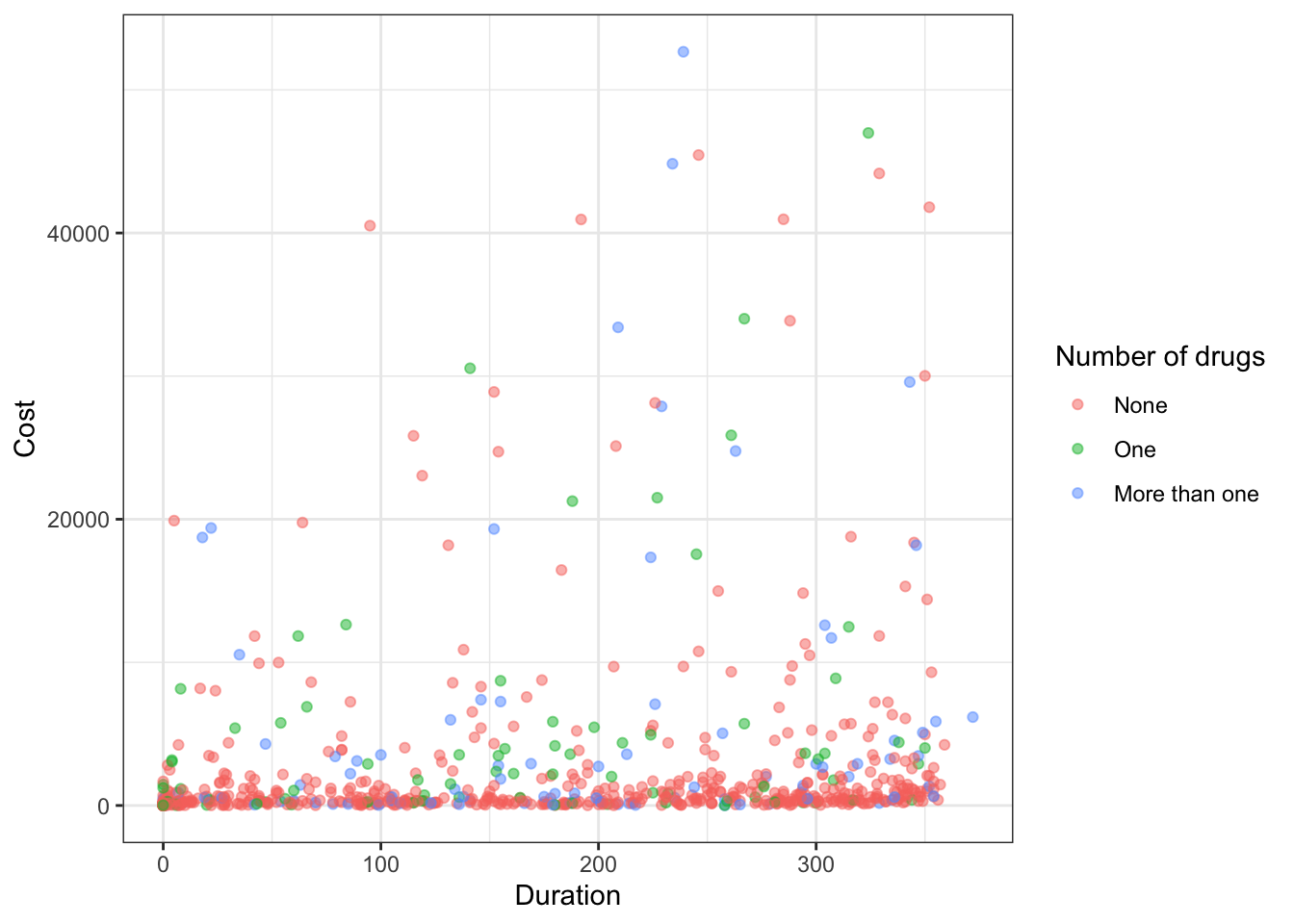What does `alpha` change? We can change the color plot for this plot using `scale_color_brewer()` function:

``````heart_disease %>%
ggplot(aes(x = Duration, y = Cost,
color = Drugs)) +
geom_point(alpha = 0.5) +
scale_color_brewer(palette = "Set2") +
labs(x = "Duration", y = "Cost",
color = "Number of drugs") +
theme_bw()``````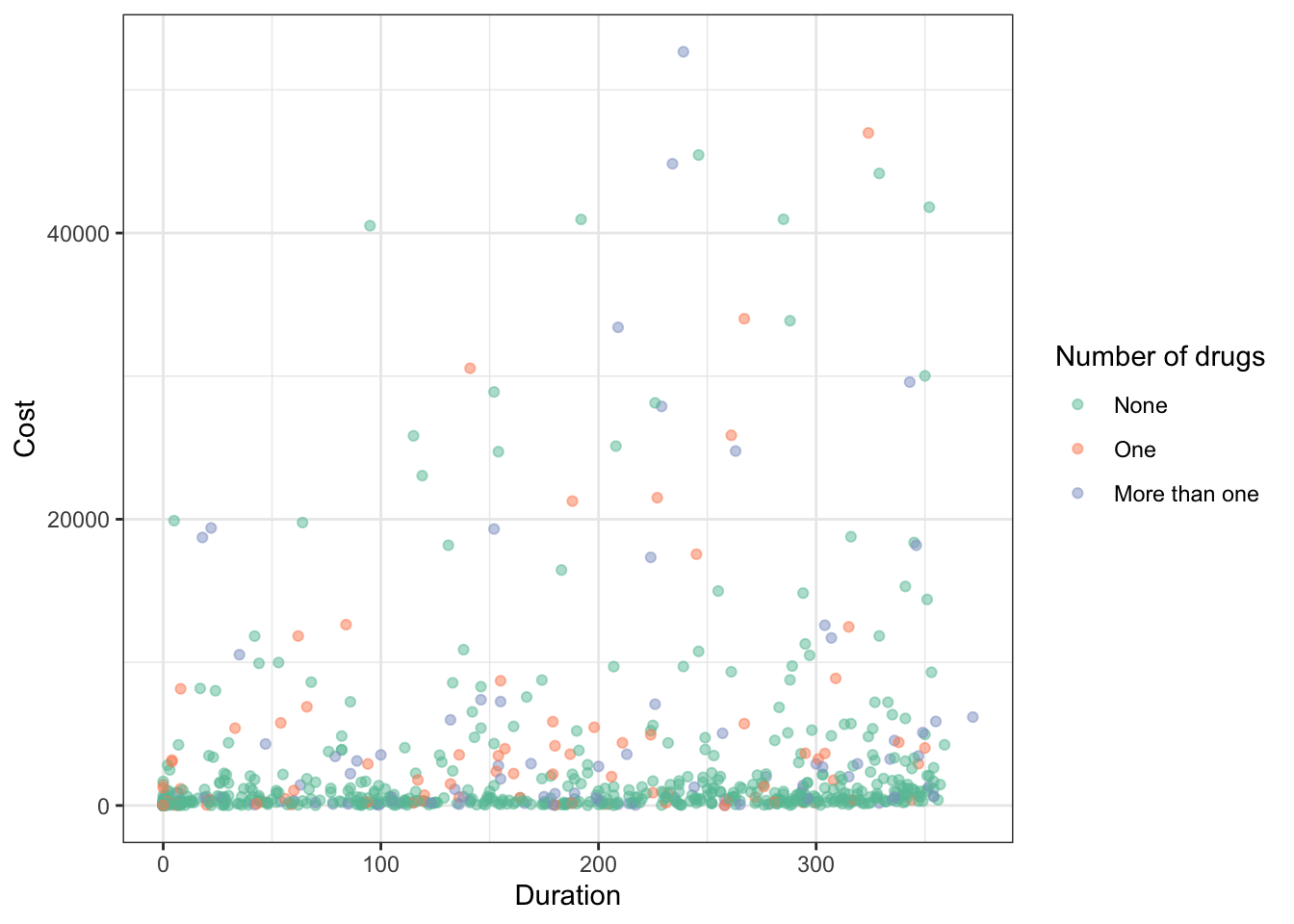#### (ALL TOGETHER NOW)

Which do you prefer, the default palette or this new one? You can check out more color palettes here.

Something you should keep in mind is to pick a color-blind friendly palette. One simple way to do this is by using the `ggthemes` package (you need to install it first before running this code!) which has color-blind friendly palettes included:

#### (SPORTS)

``````nba_stats %>%
ggplot(aes(x = offensive_rebounds, y = three_pointers,
color = position)) +
geom_point(alpha = 0.5) +
# Notice I did not use library(ggthemes) to do this... just '::'
ggthemes::scale_color_colorblind() +
labs(x = "Offensive rebounds", y = "Three-pointers",
color = "Position") +
theme_bw()``````In terms of displaying color from low to high, the viridis scales are excellent choices (and are also color-blind friendly!). For instance, we can map another continuous variable (`minutes_played`) to the color:

``````nba_stats %>%
ggplot(aes(x = offensive_rebounds, y = three_pointers,
color = minutes_played)) +
geom_point(alpha = 0.5) +
scale_color_viridis_c() +
labs(x = "Offensive rebounds", y = "Three-pointers",
color = "Minutes played") +
theme_bw()``````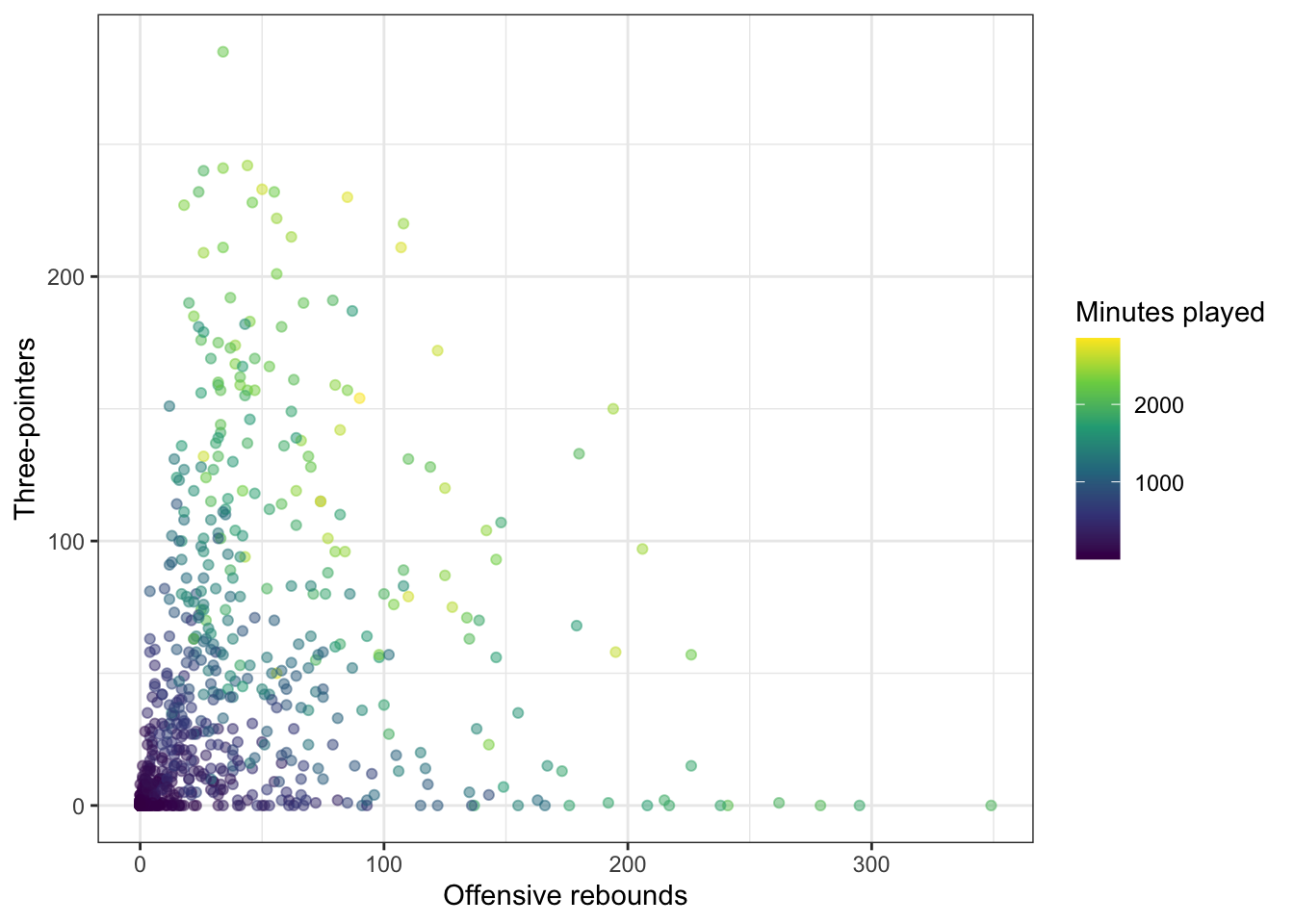#### (HEALTH)

``````heart_disease %>%
ggplot(aes(x = Duration, y = Cost,
color = Drugs)) +
geom_point(alpha = 0.5) +
# Notice I did not use library(ggthemes) to do this... just '::'
ggthemes::scale_color_colorblind() +
labs(x = "Duration", y = "Cost",
color = "Number of drugs") +
theme_bw()``````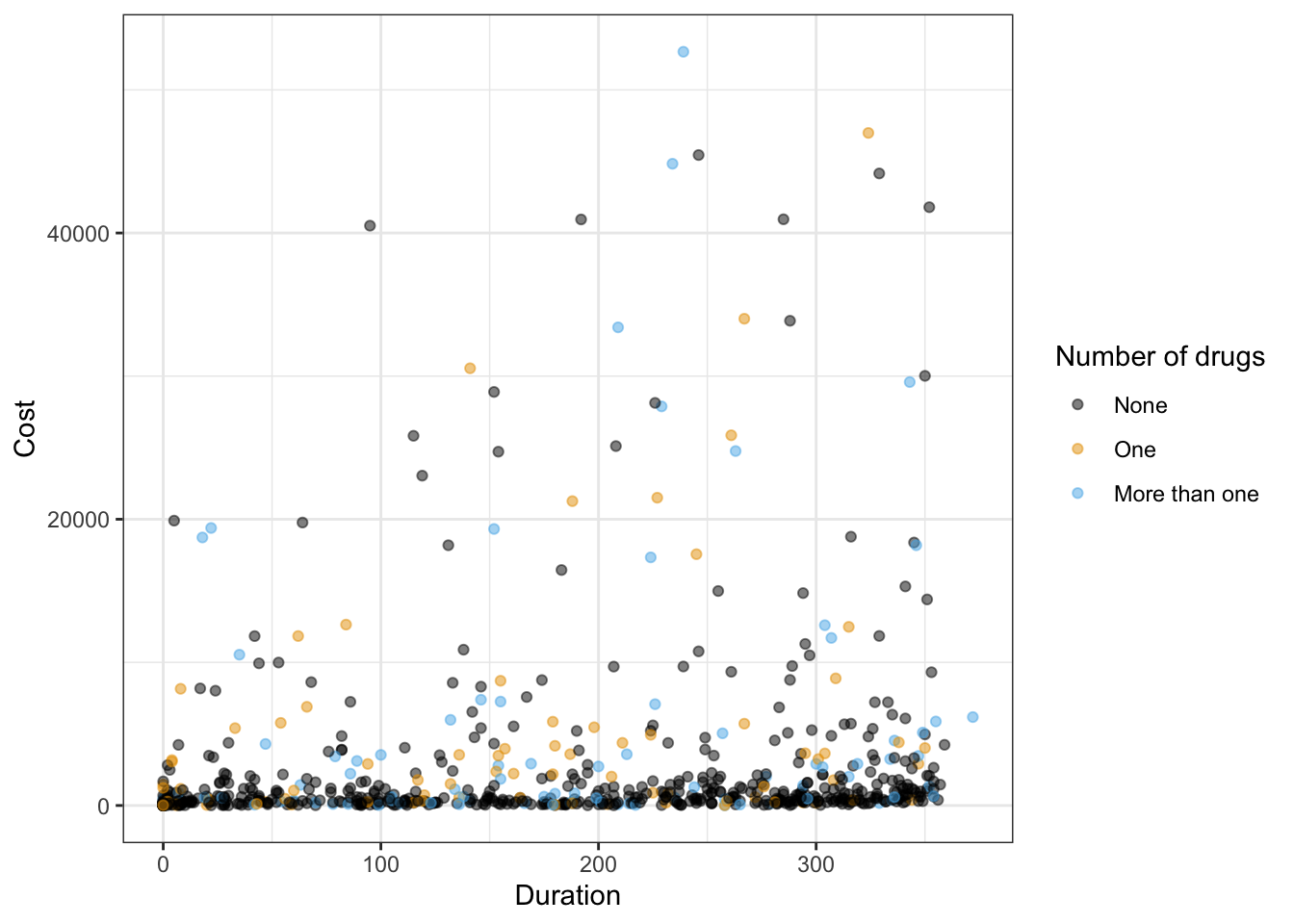In terms of displaying color from low to high, the viridis scales are excellent choices (and are also color-blind friendly!). For instance, we can map another quantitative variable (`Interventions`) to the color:

``````heart_disease %>%
ggplot(aes(x = Duration, y = Cost,
color = Interventions)) +
geom_point(alpha = 0.5) +
scale_color_viridis_c() +
labs(x = "Duration", y = "Cost",
color = "Interventions") +
theme_bw()``````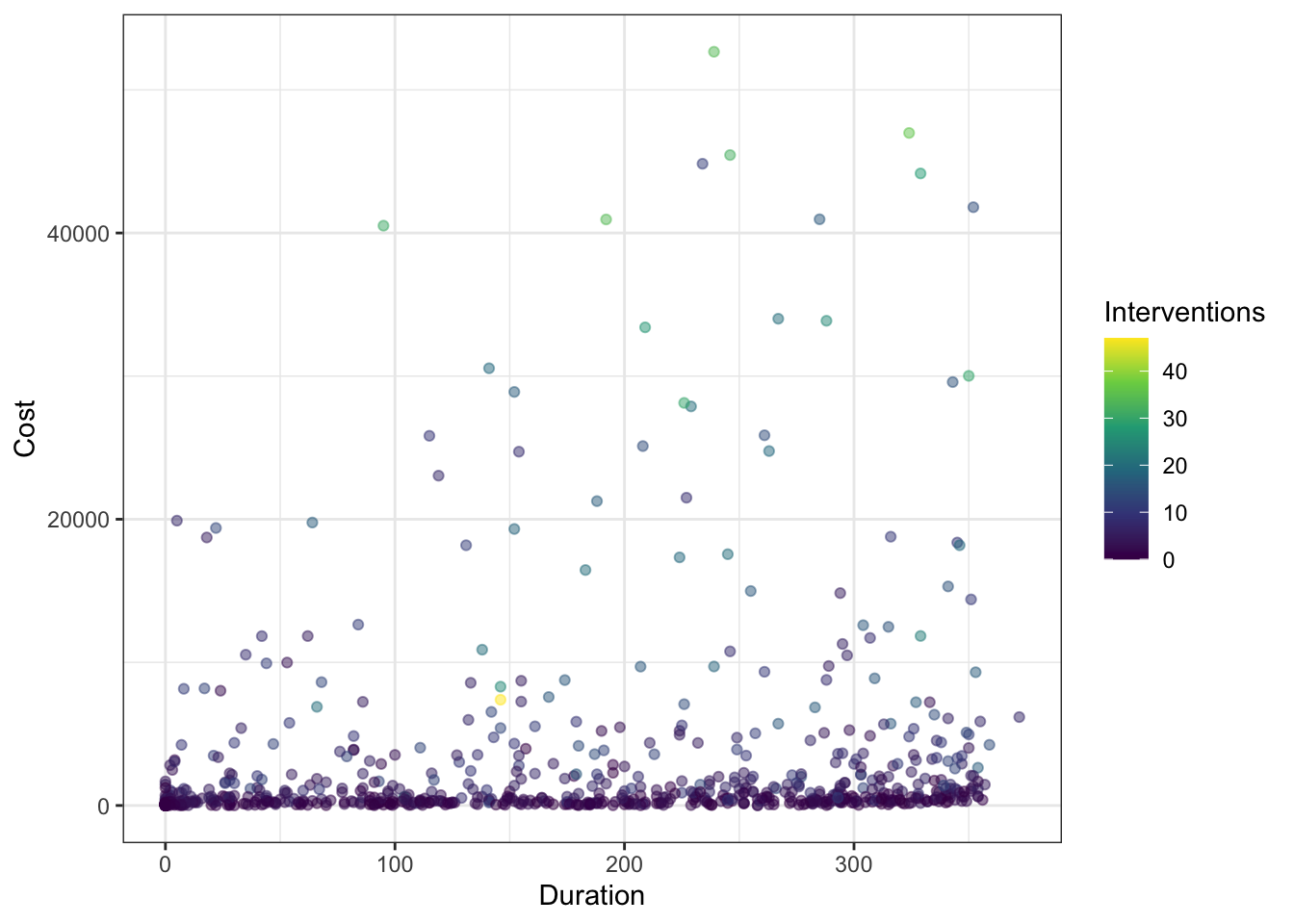#### (ALL TOGETHER NOW)

What does this reveal about the plot? What happens if you delete `scale_color_viridis_c() +` from above? Which do you prefer?

## Notes on themes

You might have noticed above have various changes to the `theme` of plots for customization. You will constantly be changing the theme of your plots to optimize the display. Fortunately, there are a number of built-in themes you can use to start with rather than the default `theme_gray()`:

#### (SPORTS)

``````nba_stats %>%
ggplot(aes(x = offensive_rebounds, y = three_pointers,
color = minutes_played)) +
geom_point(alpha = 0.5) +
scale_color_viridis_c() +
labs(x = "Offensive rebounds", y = "Three-pointers",
color = "Minutes played") +
theme_gray()``````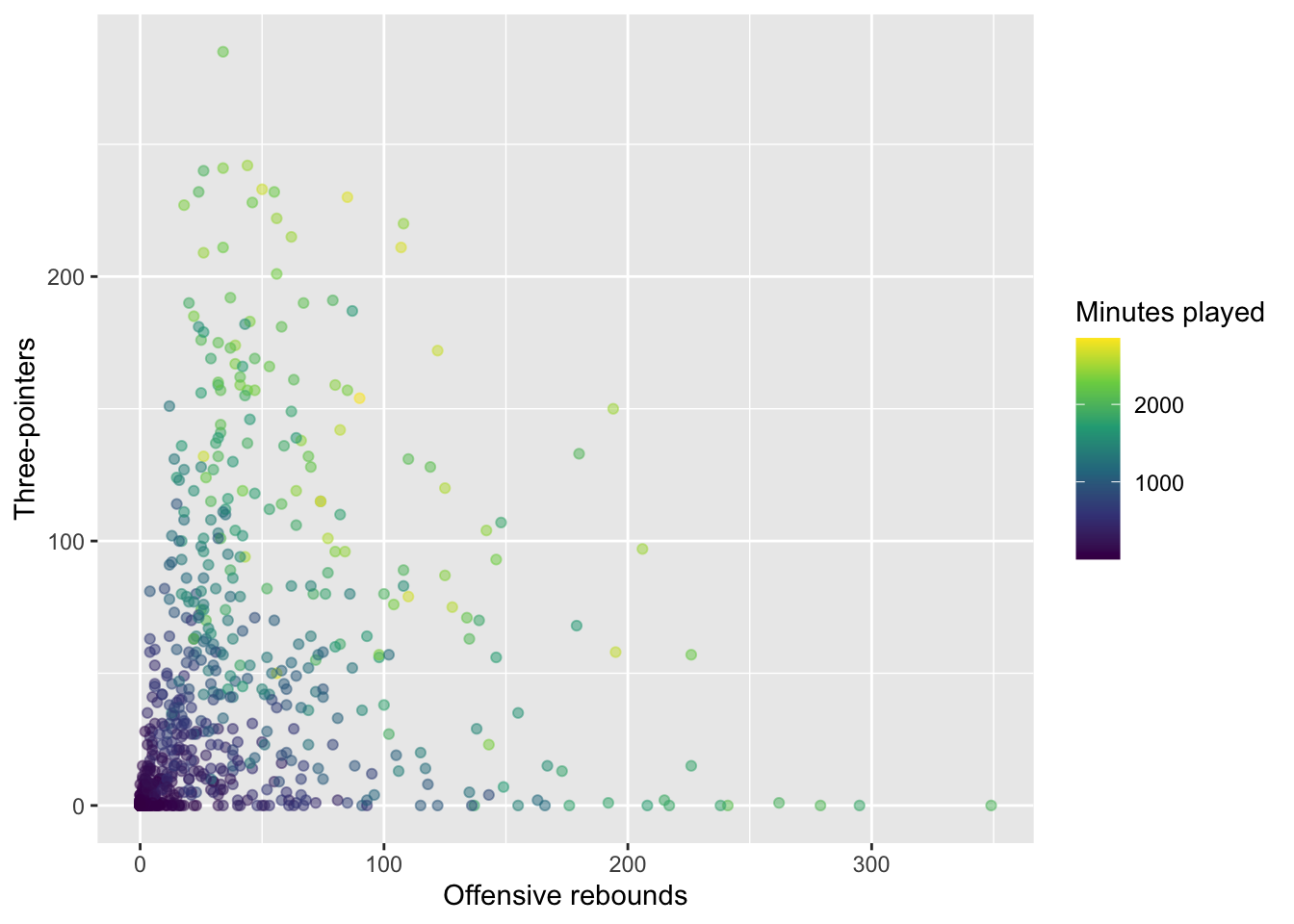For instance, Prof Yurko prefers `theme_bw()`:

``````nba_stats %>%
ggplot(aes(x = offensive_rebounds, y = three_pointers,
color = minutes_played)) +
geom_point(alpha = 0.5) +
scale_color_viridis_c() +
labs(x = "Offensive rebounds", y = "Three-pointers",
color = "Minutes played") +
theme_bw()``````There are options such as `theme_minimal()`:

``````nba_stats %>%
ggplot(aes(x = offensive_rebounds, y = three_pointers,
color = minutes_played)) +
geom_point(alpha = 0.5) +
scale_color_viridis_c() +
labs(x = "Offensive rebounds", y = "Three-pointers",
color = "Minutes played") +
theme_minimal()``````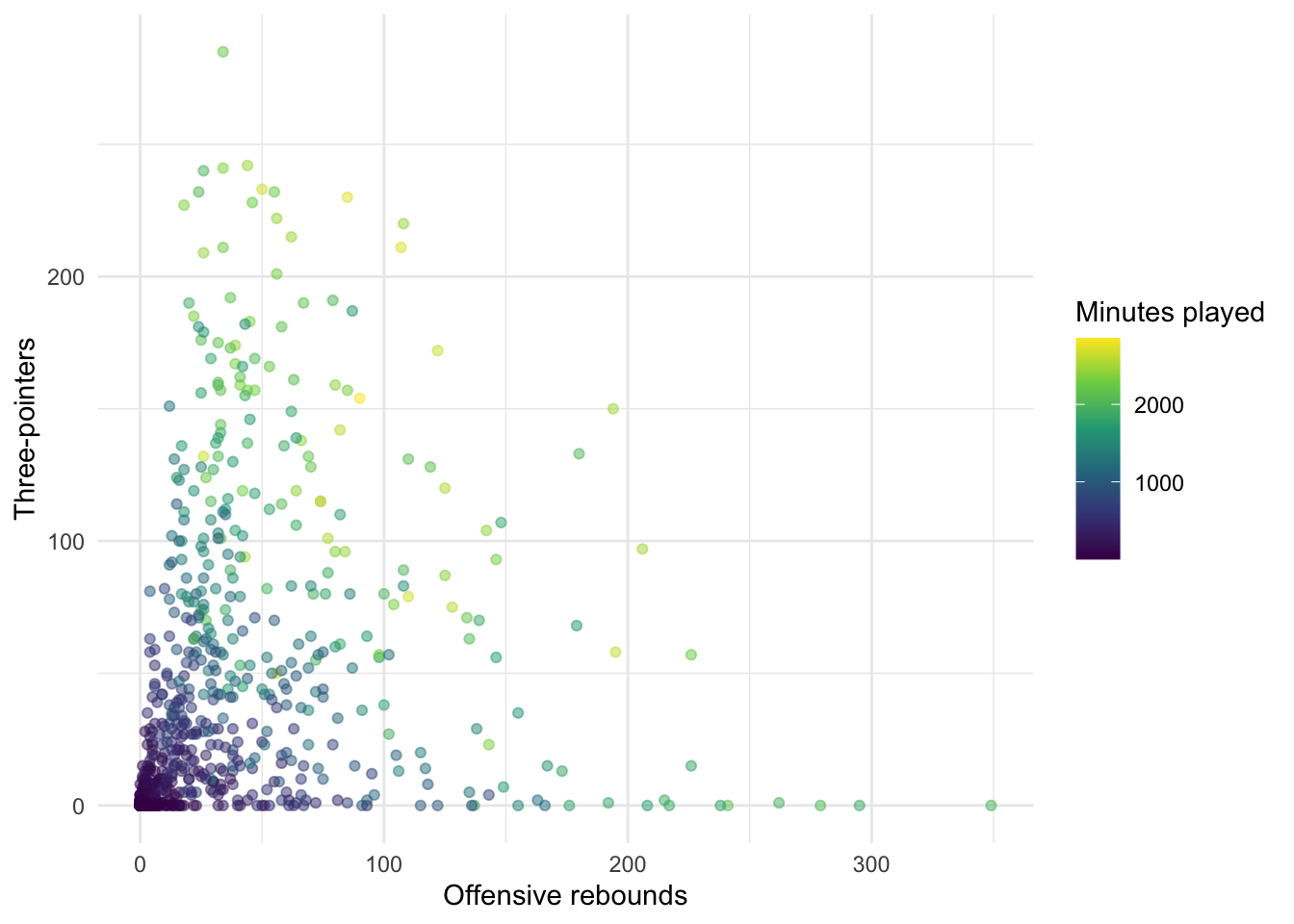or `theme_classic()`:

``````nba_stats %>%
ggplot(aes(x = offensive_rebounds, y = three_pointers,
color = minutes_played)) +
geom_point(alpha = 0.5) +
scale_color_viridis_c() +
labs(x = "Offensive rebounds", y = "Three-pointers",
color = "Minutes played") +
theme_classic()``````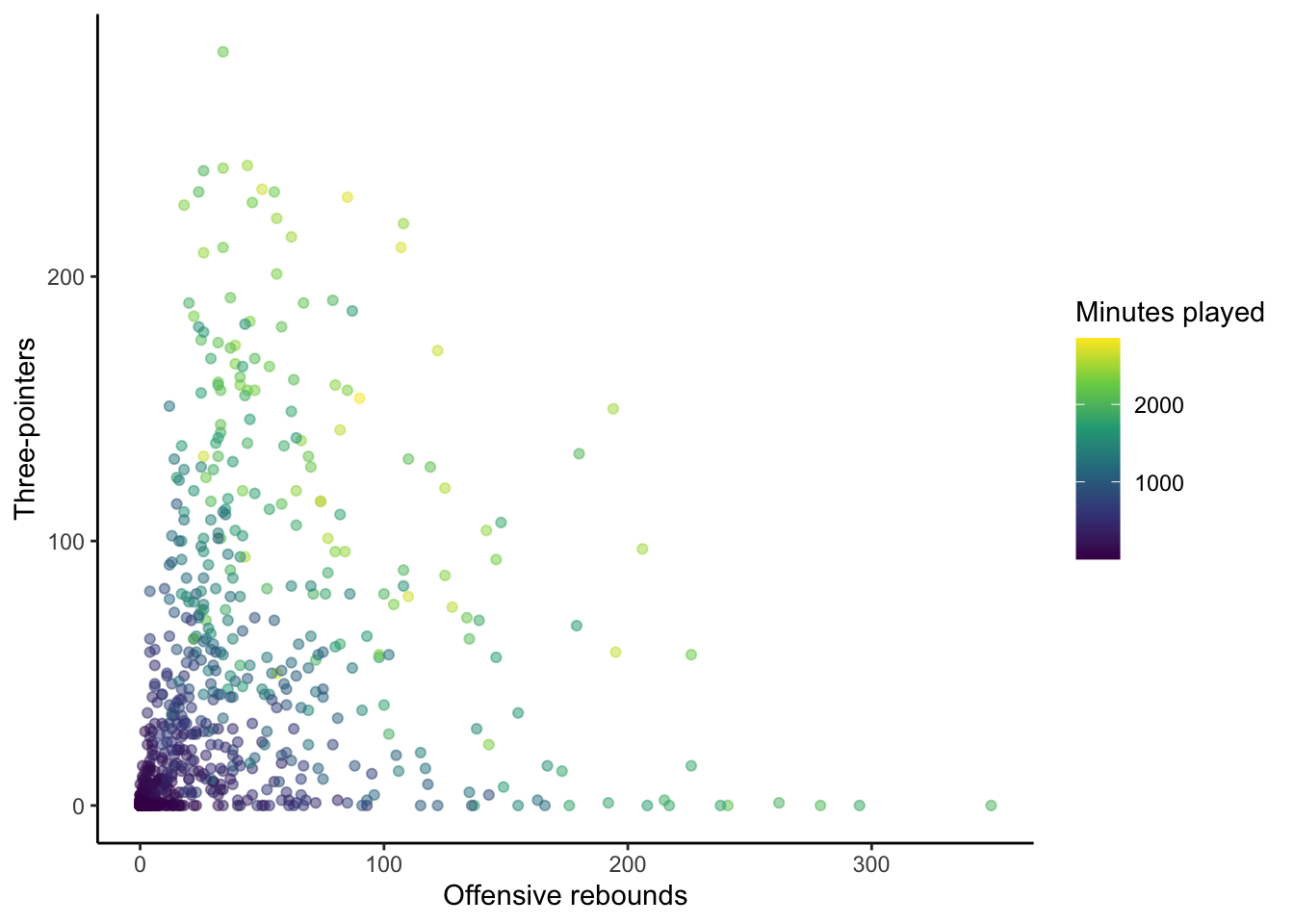There are also packages with popular themes, such as the `ggthemes` package which includes, for example, `theme_economist()`:

``````library(ggthemes)
nba_stats %>%
ggplot(aes(x = offensive_rebounds, y = three_pointers,
color = minutes_played)) +
geom_point(alpha = 0.5) +
scale_color_viridis_c() +
labs(x = "Offensive rebounds", y = "Three-pointers",
color = "Minutes played") +
theme_economist()``````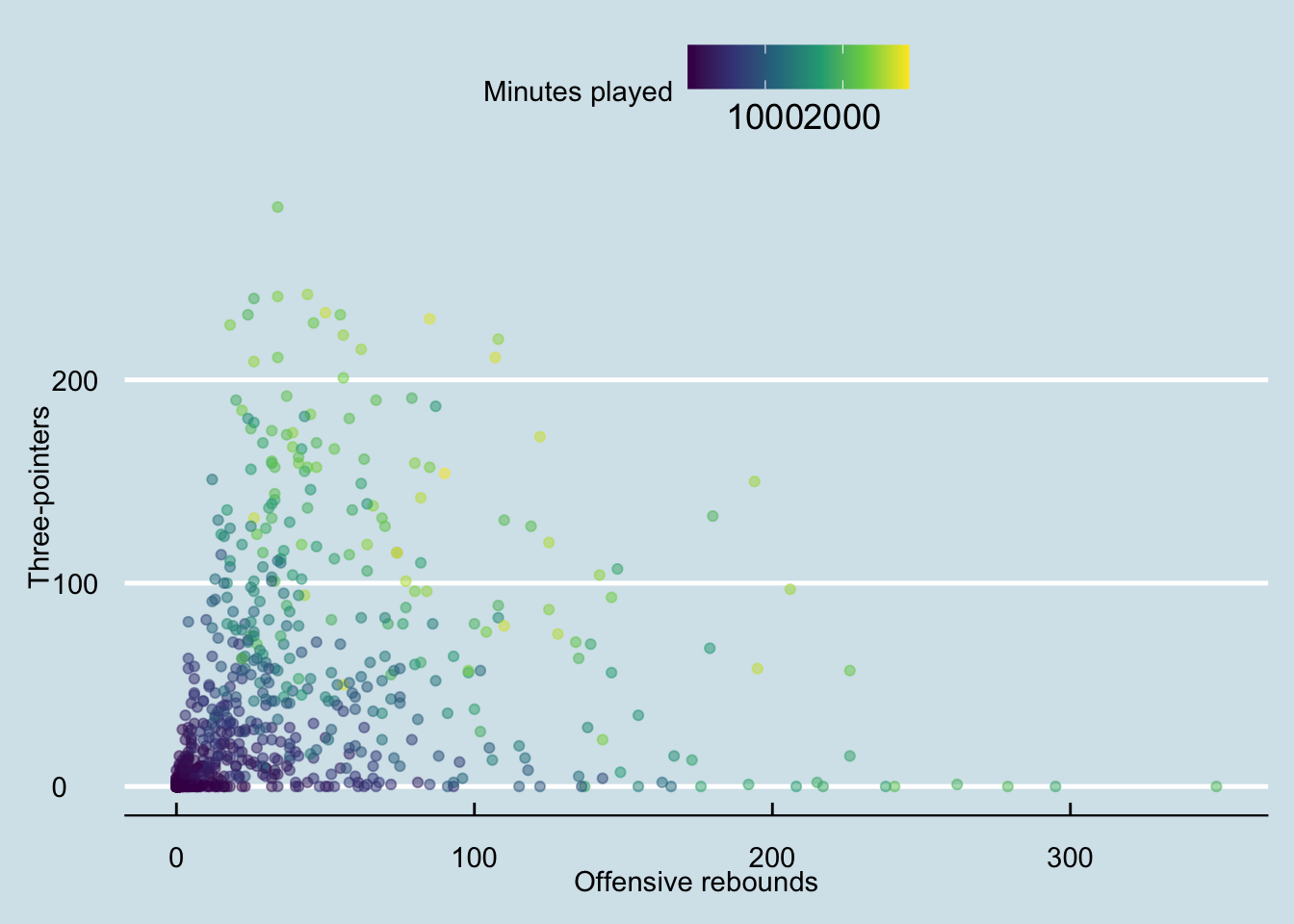and `theme_fivethirtyeight()` to name a couple:

``````nba_stats %>%
ggplot(aes(x = offensive_rebounds, y = three_pointers,
color = minutes_played)) +
geom_point(alpha = 0.5) +
scale_color_viridis_c() +
labs(x = "Offensive rebounds", y = "Three-pointers",
color = "Minutes played") +
theme_fivethirtyeight()``````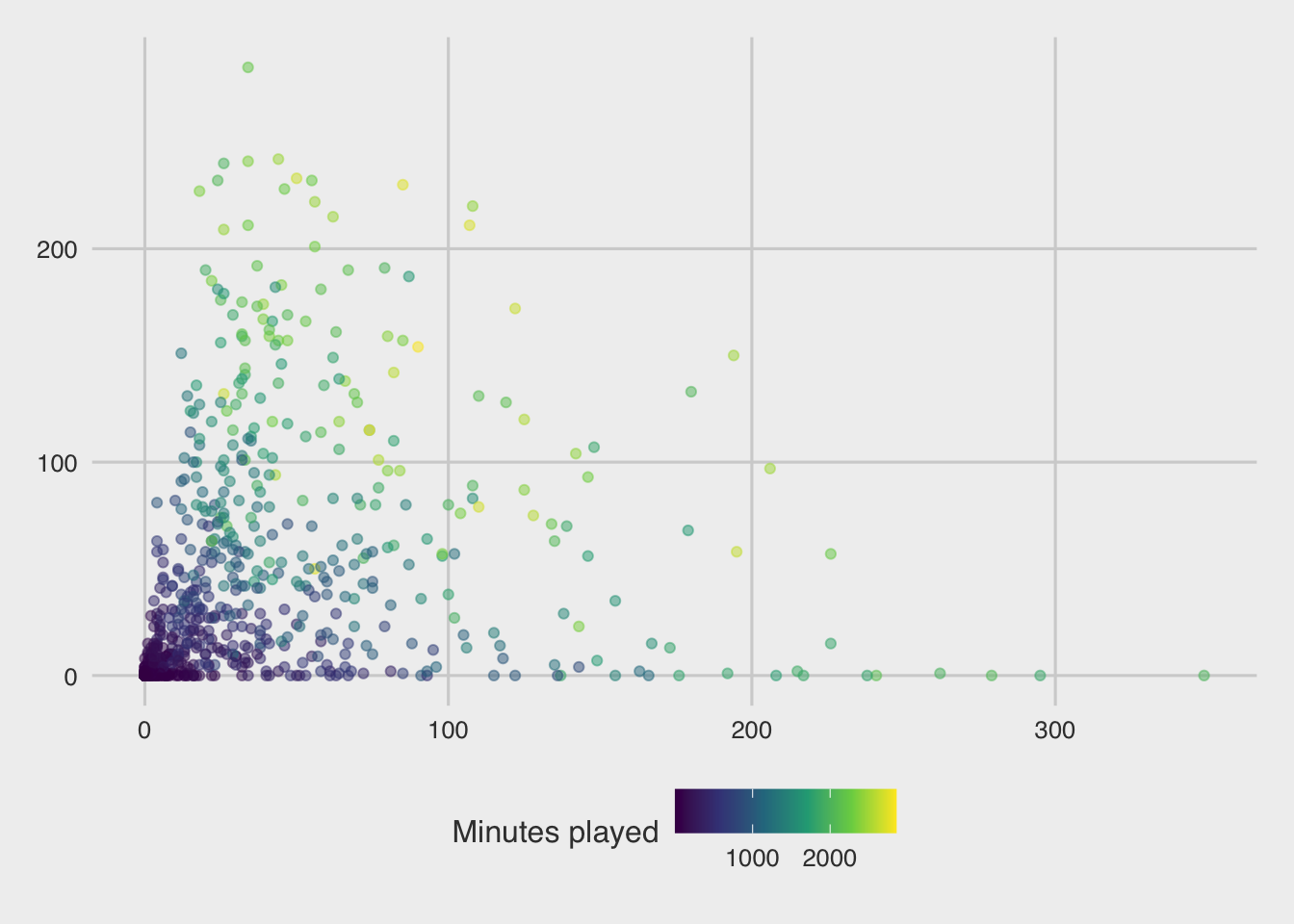With any theme you have picked, you can then modify specific components directly using the `theme()` layer. There are many aspects of the plot’s theme to modify, such as my decision to move the legend to the bottom of the figure, drop the legend title, and increase the font size for the y-axis:

``````nba_stats %>%
ggplot(aes(x = offensive_rebounds, y = three_pointers,
color = minutes_played)) +
geom_point(alpha = 0.5) +
scale_color_viridis_c() +
labs(x = "Offensive rebounds", y = "Three-pointers",
title = "Joint distribution of three-pointers and offensive rebounds",
subtitle = "NBA statistics from 2021-2022 season",
color = "Minutes played") +
theme_bw() +
theme(legend.position = "bottom",
legend.title = element_blank(),
axis.text.y = element_text(size = 14),
axis.text.x = element_text(size = 6))``````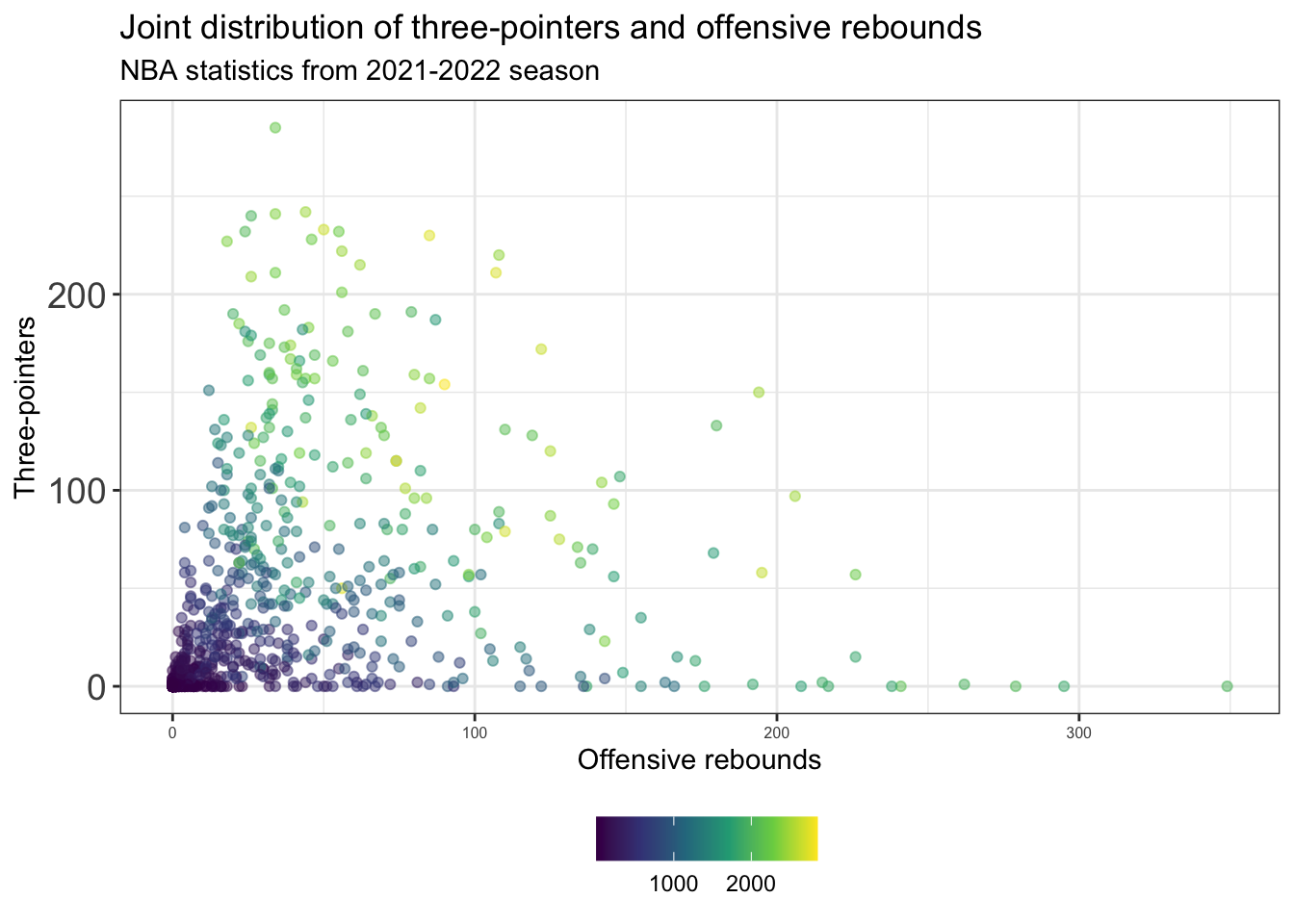#### (HEALTH)

Starting with the default `theme_gray()`:

``````heart_disease %>%
ggplot(aes(x = Duration, y = Cost,
color = Interventions)) +
geom_point(alpha = 0.5) +
scale_color_viridis_c() +
labs(x = "Duration", y = "Cost",
color = "Interventions") +
theme_gray()``````Many other themes are out there. For instance, Prof Yurko prefers `theme_bw()`:

``````heart_disease %>%
ggplot(aes(x = Duration, y = Cost,
color = Interventions)) +
geom_point(alpha = 0.5) +
scale_color_viridis_c() +
labs(x = "Duration", y = "Cost",
color = "Interventions") +
theme_bw()``````There are options such as `theme_minimal()`:

``````heart_disease %>%
ggplot(aes(x = Duration, y = Cost,
color = Interventions)) +
geom_point(alpha = 0.5) +
scale_color_viridis_c() +
labs(x = "Duration", y = "Cost",
color = "Interventions") +
theme_minimal()``````or `theme_classic()`:

``````heart_disease %>%
ggplot(aes(x = Duration, y = Cost,
color = Interventions)) +
geom_point(alpha = 0.5) +
scale_color_viridis_c() +
labs(x = "Duration", y = "Cost",
color = "Interventions") +
theme_classic()``````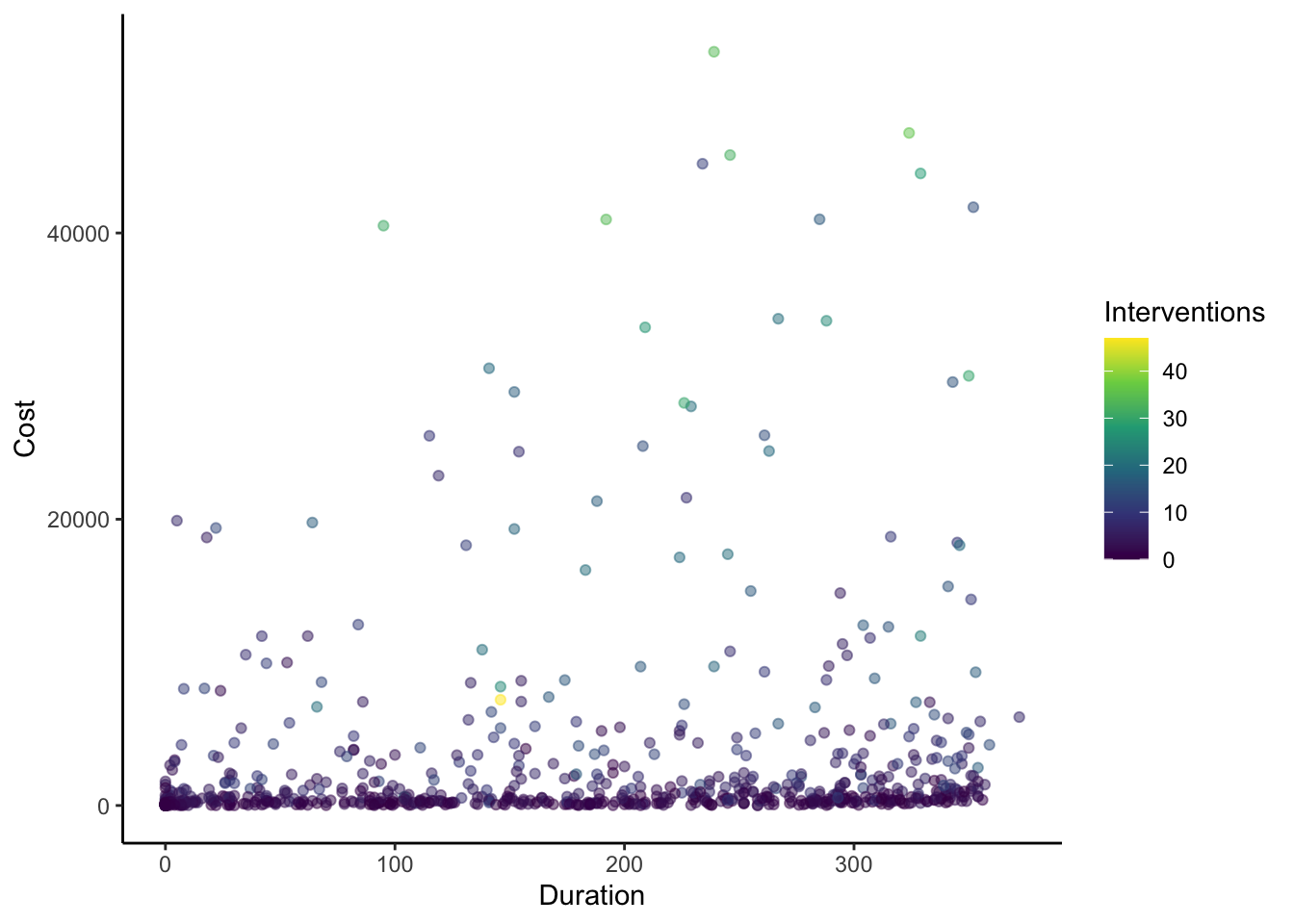There are also packages with popular themes, such as the `ggthemes` package which includes, for example, `theme_economist()`:

``````library(ggthemes)
heart_disease %>%
ggplot(aes(x = Duration, y = Cost,
color = Interventions)) +
geom_point(alpha = 0.5) +
scale_color_viridis_c() +
labs(x = "Duration", y = "Cost",
color = "Interventions") +
theme_economist()``````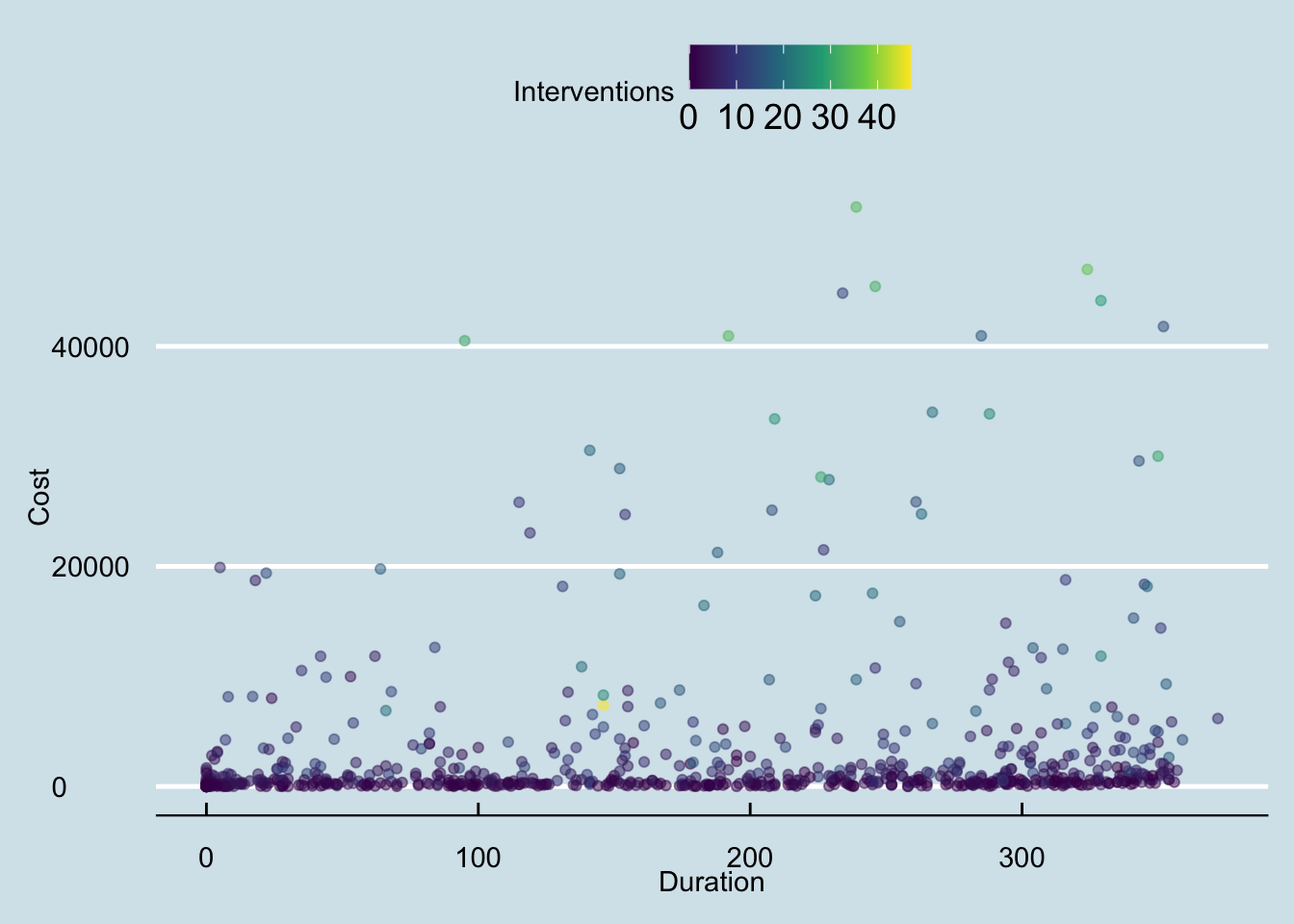and `theme_fivethirtyeight()` to name a couple:

``````heart_disease %>%
ggplot(aes(x = Duration, y = Cost,
color = Interventions)) +
geom_point(alpha = 0.5) +
scale_color_viridis_c() +
labs(x = "Duration", y = "Cost",
color = "Interventions") +
theme_fivethirtyeight()``````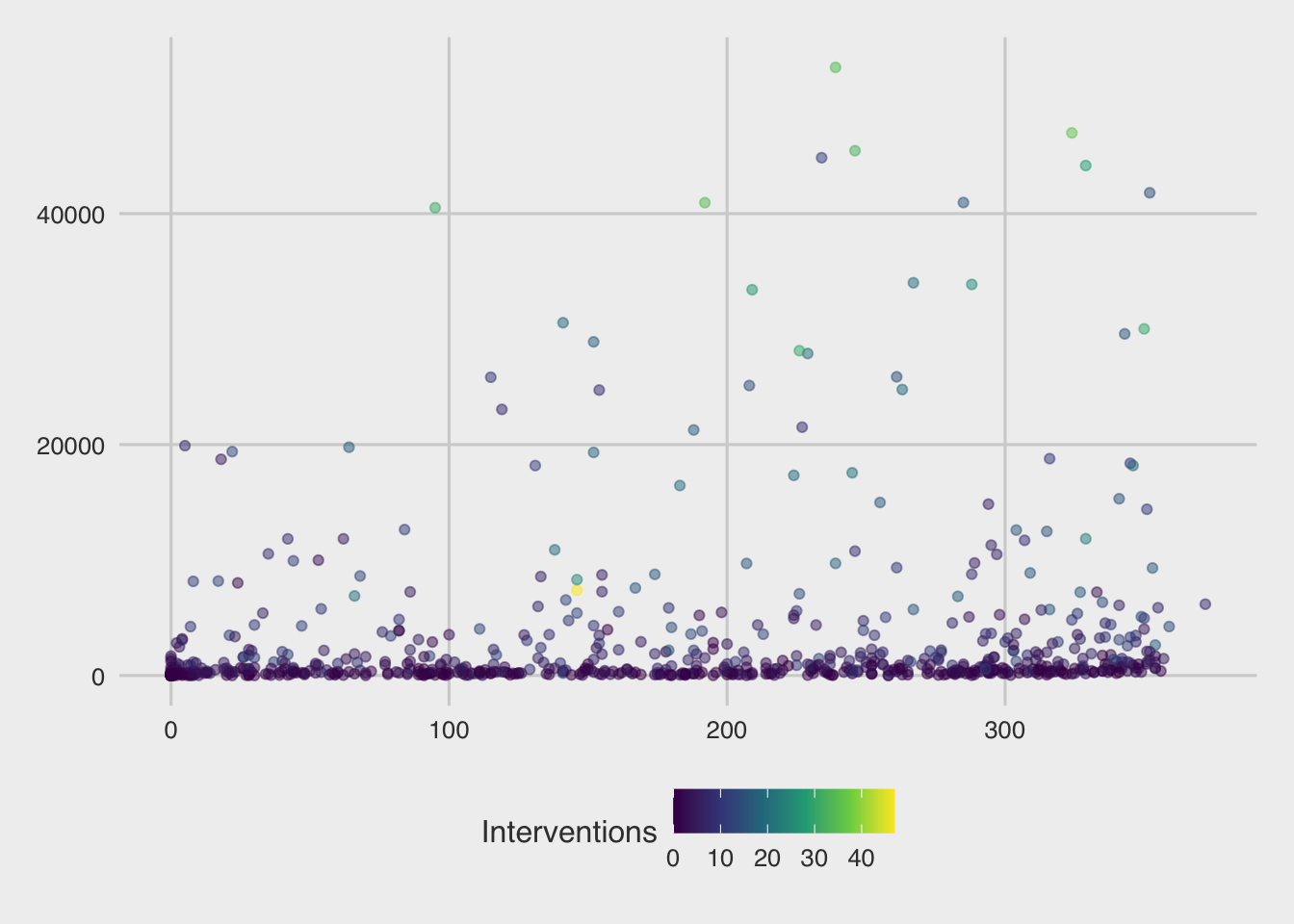With any theme you have picked, you can then modify specific components directly using the `theme()` layer. There are many aspects of the plot’s theme to modify, such as my decision to move the legend to the bottom of the figure, drop the legend title, and increase the font size for the y-axis:

``````heart_disease %>%
ggplot(aes(x = Duration, y = Cost,
color = Interventions)) +
geom_point(alpha = 0.5) +
scale_color_viridis_c() +
labs(x = "Duration", y = "Cost",
title = "Joint distribution of patients' duration and cost",
color = "Interventions") +
theme_bw() +
theme(legend.position = "bottom",
legend.title = element_blank(),
axis.text.y = element_text(size = 14),
axis.text.x = element_text(size = 6))``````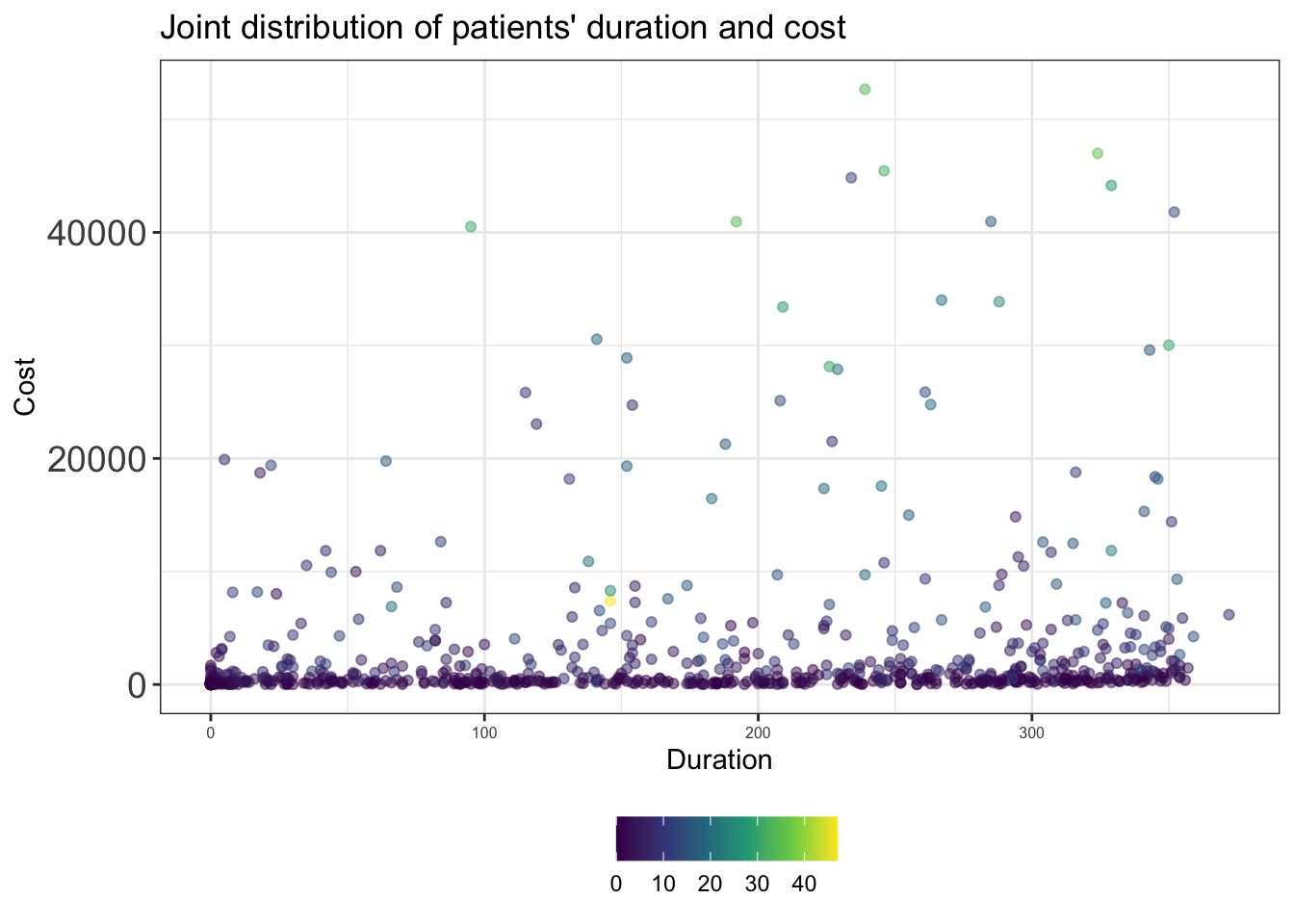#### (ALL TOGETHER NOW)

If you’re tired of explicitly customizing every plot in the same way all the time, then you should make a custom theme. It’s quite easy to make a custom theme for `ggplot2` and of course there are an incredible number of ways to customize your theme. In the code chunk, I modify the `theme_bw()` theme using the `%+replace%` argument to make my new theme named `my_theme()` - which is stored as a function:

``````my_theme <- function () {
theme_bw(base_size = 10) %+replace%
theme(
panel.background  = element_blank(),
plot.background = element_rect(fill = "transparent", color = NA),
legend.position = "bottom",
legend.background = element_rect(fill = "transparent", color = NA),
legend.key = element_rect(fill = "transparent", color = NA),
axis.ticks = element_blank(),
panel.grid.major = element_line(color = "grey90", size = 0.3),
panel.grid.minor = element_blank(),
plot.title = element_text(size = 18,
hjust = 0, vjust = 0.5,
face = "bold",
margin = margin(b = 0.2, unit = "cm")),
plot.subtitle = element_text(size = 12, hjust = 0,
vjust = 0.5,
margin = margin(b = 0.2, unit = "cm")),
plot.caption = element_text(size = 7, hjust = 1,
face = "italic",
margin = margin(t = 0.1, unit = "cm")),
axis.text.x = element_text(size = 13),
axis.text.y = element_text(size = 13)
)
}``````

Now I can go ahead and make my plot from before with this theme:

#### (SPORTS)

``````nba_stats %>%
ggplot(aes(x = offensive_rebounds, y = three_pointers,
color = minutes_played)) +
geom_point(alpha = 0.5) +
scale_color_viridis_c() +
labs(x = "Offensive rebounds", y = "Three-pointers",
title = "Joint distribution of three-pointers and offensive rebounds",
subtitle = "NBA statistics from 2021-2022 season",
color = "Minutes played") +
my_theme()``````
``````Warning: The `size` argument of `element_line()` is deprecated as of ggplot2 3.4.0.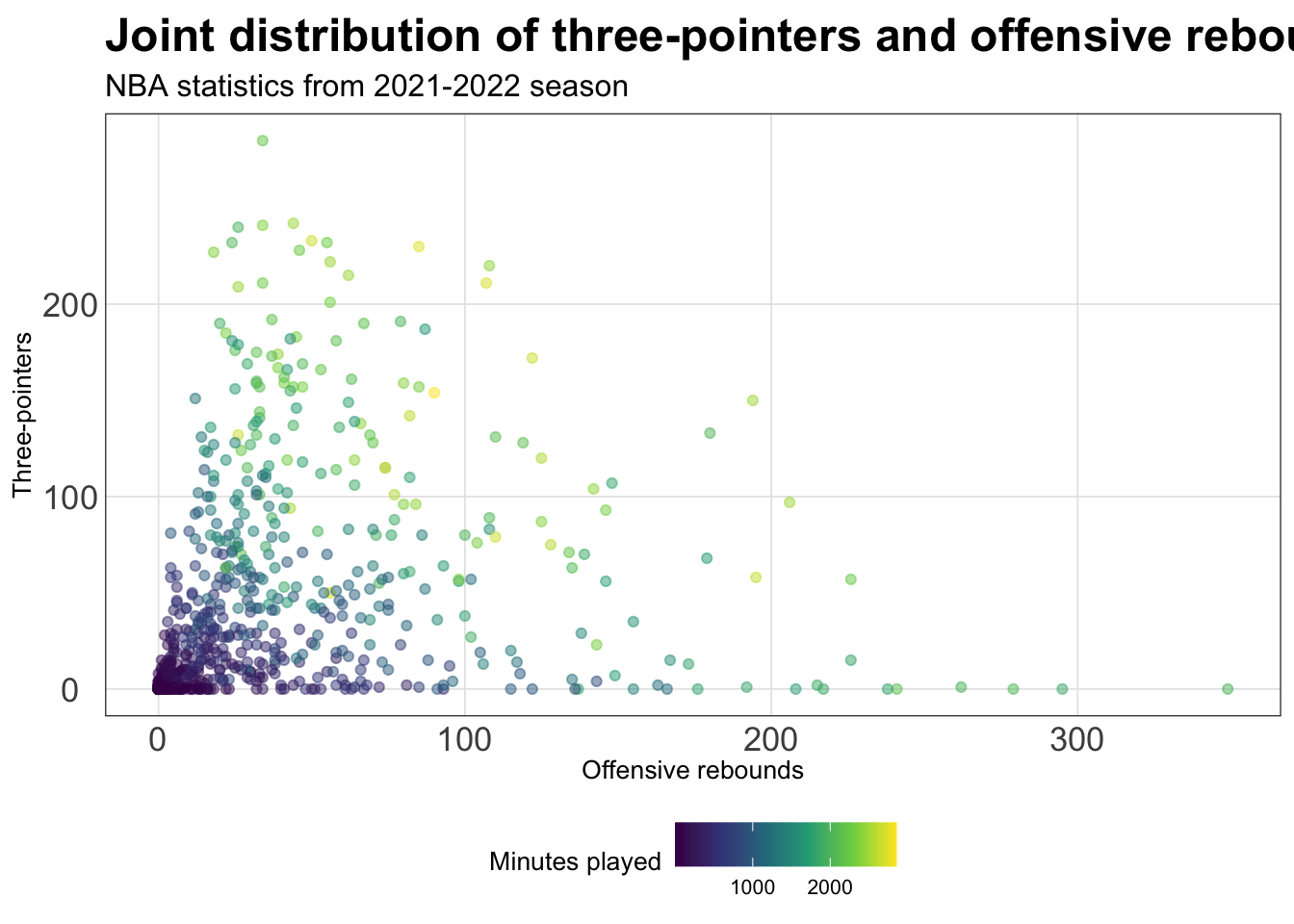#### (HEALTH)

``````heart_disease %>%
ggplot(aes(x = Duration, y = Cost,
color = Interventions)) +
geom_point(alpha = 0.5) +
scale_color_viridis_c() +
labs(x = "Duration", y = "Cost",
title = "Joint distribution of patients' duration and cost",
color = "Interventions") +
my_theme()``````